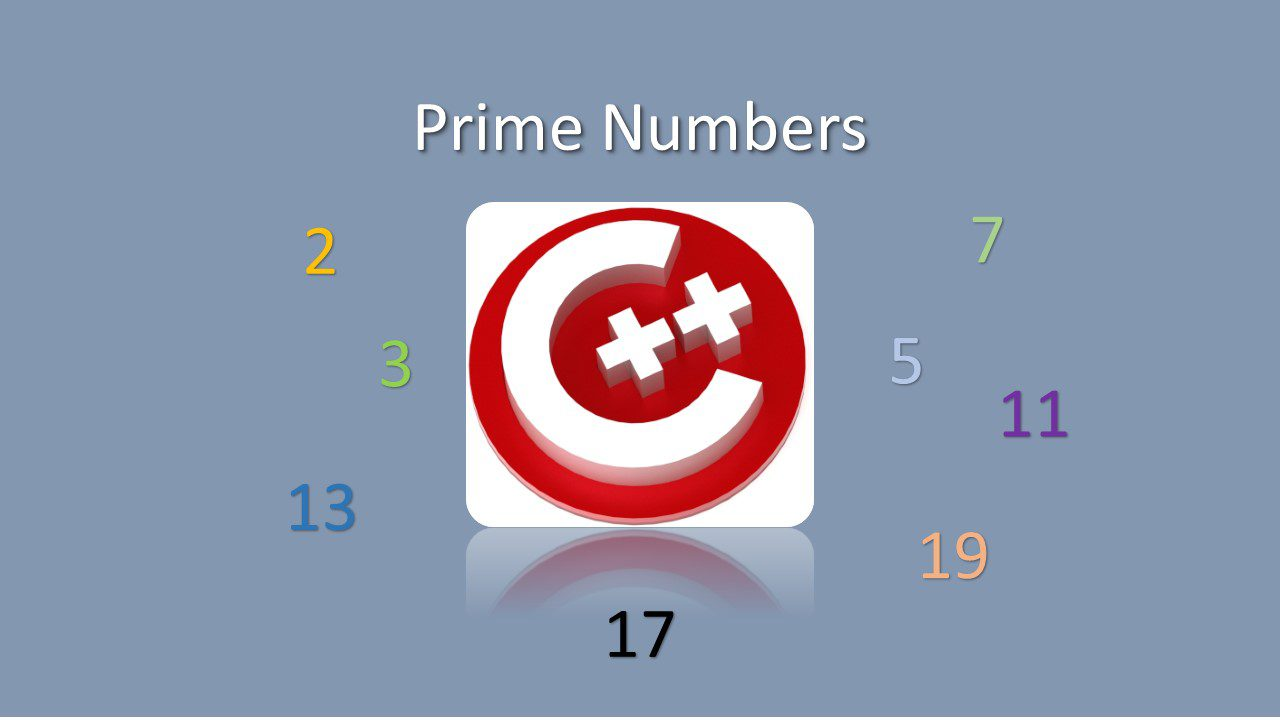# Easily Learn To Find Prime Numbers In Modern C++Prime numbers are interesting area to research. A prime number, it is also called prime shortly , is a natural number (a positive integer)  greater than 1 that is not a product of two smaller natural numbers. If a number is not prime then it is called as a composed number. There are several mathematical questions regarding prime numbers are still unsolved.  Finding them and relations and their effects on some other functions, graphics really interesting in mathematical phenomena.

Calculating prime numbers in a range is another comparison in programming languages. C++ is the fastest and most powerful programming language and it is easy to calculate prime numbers. In this post, we will explain how to find Prime Numbers in C++ Builder. C++ Builder is a great IDE, includes compilers for Windows, Android and iOS. C++Builder has both CLANG Enhanced C/C++ Compiler and a Borland C/C++ Compiler. You can download the free C++ Builder Community Edition here: https://www.embarcadero.com/products/cbuilder/starter. Professional developers can use the Professional, Architect or Enterprise versions of C++ Builder. Please visit https://www.embarcadero.com/products/cbuilder.

Now, lets start to find if a given number is prime or not. To do this, we will create our own prime number .

This function is counting all number and may be slower, we don’t need to count all of them. Trial division is the most laborious but easiest to understand of the integer factorization algorithms, it is well used to define maximum search number of prime numbers.. We can enhance and speed up this procedure by this method. We limit our for loop with q variable which is calculated with this method. By adding this, it will be as below;

We can say that this procedure above is the most simple to calculate if a number is a prime number or not. Note that unsigned integer ranges 0 to 4,294,967,295. For larger number you need to use double long or you may need more specific procedures for more higher numbers.

Now lets count all numbers in a given range (from 2 to maxN) by using our is_prime() function.

Finally, we can easily calculate number of primes in a given range as below;

In summary, create a new Multi-Device C++ Builder Project in C++ Builder, add a Button and a Memo components to see output. All all codes should be as below.